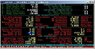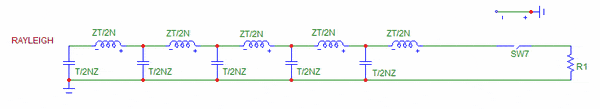# Calculating Inductance Gradient for EM Launcher

• axi0m

#### axi0m

The equation widely used to calculate the force on a projectile in an electromagnetic launcher, more specifically a railgun, is:

F = 0.5 * L' * I^2

where:
--> F is the force in Newtons
--> L-prime is the inductance gradient of the rails in henries/meter (H/m)
--> and I is the current through the rails in amperes.

How would I calculate or estimate the inductance of a given pair of rails? FYI, they are 0.0625 inches wide, 6.0 inches long and 0.25 inches high, being separated by 0.125 inches.

I have had only a brief introduction to calculus, therefore I'm not entirely sure what to do with the "L-prime" factor, which is measured in henries/meter. I know that inductance is measured in henries. Would I simply take the total inductance of the rails, in henries, and divide this by the length of the rails, in meters? If so, why is the considered "prime?" It seems that I remember when something was prime we applied the "power rule" to it, in my former calculus class, that is.

Any help would be GREATLY appreciated. Thanks in advance for your time.

I'll just go ahead and throw this out there. I played around with some numbers, maybe someone could at least tell me if this makes sense to them.

I've read a few places that a common inductance gradient for a simple railgun is 0.5 H/m.

Given the equation:

F = 0.5 * L' * I^2

if I plug 0.5 in for L, would the derivative of that be 2.0?

If I have a current pulse of roughly 20,000 amperes then,

0.5 * 2.0 * 20,000^2

would yield 4e8, or 400,000,000.00 Newtons of force on the projectile.

Sigh.

I think that inductance figure would be much too high. A Henry is actually quite a big unit.
I'd be surprised if your rail has an inductance of more than 5 microhenries / meter.

Don't know if it would help, but I am attaching a great program that is intended for use with transmission lines. It was written by Reg Edwards G4FGQ, now deceased.

You will need to work out the Characteristic Impedance of your line.
Z = 276 log (2 * (distance between lines, centre to centre) / (diameter of conductor ))
Units just have to be the same.

My best estimate was 131 ohms.

I ran this program and I get an Inductance of 0.44 uH per meter using a shorted end on the rail. You might like to try it. I had to guess a bit with the input data. :)

#### Attachments

• rjeline4.zip
34.6 KB · Views: 356
•rail gun 2.JPG
63.1 KB · Views: 661
I think that inductance figure would be much too high. A Henry is actually quite a big unit.
I'd be surprised if your rail has an inductance of more than 5 microhenries / meter.

Don't know if it would help, but I am attaching a great program that is intended for use with transmission lines. It was written by Reg Edwards G4FGQ, now deceased.

You will need to work out the Characteristic Impedance of your line.
Z = 276 log (2 * (distance between lines, centre to centre) / (diameter of conductor ))
Units just have to be the same.

My best estimate was 131 ohms.

I ran this program and I get an Inductance of 0.44 uH per meter using a shorted end on the rail. You might like to try it. I had to guess a bit with the input data. :)
Yes, looking back my mistake was using .5H instead of .5uH. Foolish me!

By now, I've changed plans a bit. I'm looking to create said electromagnetic launcher with more than one pair of rails, four to be exact. I'm not sure if I could still use that program for this. Below is a cross-section of the rail geometry.

http://img195.imageshack.us/img195/4302/railgun.png [Broken]

The gray box in the middle is the 0.25" by 0.25" bore of the projectile. The rails are all 0.61 meters long. Do you know if I could use that same program to calculate the inductance gradient of this rail geometry? If not, is there any way to exactly calculate it?

I understand this is fairly similar to transmission line theory, but my knowledge of that is limited to a few hours of reading on the internet. I'm hoping the inductance gradient of this rail geometry is somewhere between 5uH/m and 7uH/m. If so, the system efficiency could be as high as 60%.

Last edited by a moderator:
That program is only for one pair of wires but you could probably work out something if the rails are all identical pairs, as they probably would be.

This would depend on how you connected the rails and how much coupling there was between them.

What is your exact setup though? How are you going to power this rail gun and how are you going to distribute the power on the 8 rails?

That program is only for one pair of wires but you could probably work out something if the rails are all identical pairs, as they probably would be.

This would depend on how you connected the rails and how much coupling there was between them.

What is your exact setup though? How are you going to power this rail gun and how are you going to distribute the power on the 8 rails?

Pardon the terrible representation, but here is the general wiring scheme. And yes, they are all identical pairs, except for the two inner-most rails which I've enlarged to help decrease the current density on the sliding contact surfaces where the armature projectile will travel.

As for power, I have 10 450V 6800uF aluminum electrolytic capacitors. They have an amazingly low 25 milliohms of ESR. As such, the ten of them in parallel have an effective 2.5 milliohm ESR, making the bank capable of a 180kA pulse.

I, however, will have them set up in a Pulse Forming Network (PFN,) specifically a Rayleigh PFN. I should be able to achieve a nice square, lower-current longer-output pulse this way. Below is a schematic of the general Rayleign PFN design. I'm hoping I can make the PFN work, considering the fact that the rails will have a high inductance and might throw the PFN circuit out of wack.Last edited by a moderator: